omG8HI9LSKFW6lhCj8prTs0Z6lUhdOT9Jhi1Sf4m

# Electric Circuit Theorems and Linearity Circuit

A major advantage of analyzing circuit using Kirchhoff's laws as we did before is that we can analyze a circuit without tampering with its original configuration. The major disadvantage is that, for a large, complex circuit, tedious computation is involved.
The growth in areas of application of electric circuits has led to an evolution from simple to complex circuits. To handle the complexity, engineers over the years have developed some theorems to simplify circuit analysis.

Such theorems include :
Since these theorems are applicable to linear circuit, we first discuss the concept of circuit linearity.
In addition to circuit theorems, we discuss the concepts of:

## Linearity Property

Linearity is the property of an element describing a linear relationship between cause and effect. Although the property applies to many circuit elements, we shall limit its applicability to resistors this time. The property is a combination of both the homogeneity (scaling) property and the additivity property.

The homogeneity property requires that if the input (also called the excitation) is multiplied by a constant, then the output (also called the response) is multiplied by the same constant. For a resistor, for example, Ohm's law relates the input i to the output v,(1)
If the current is increased by a constant k, then the voltage increases corresponding by k; that is,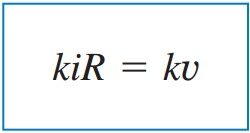(2)
The additivity property requires that the response to a sum of inputs is the sum of the responses to each input applied separately. Using the voltage-current relationship of a resistor, if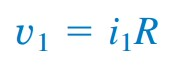(3a)
and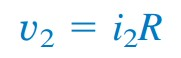(3b)
then applying (i1 + i2) gives(4)
We say that a resistor is a linear element because the voltage-current relationship satisfies both of the homogeneity and the additivity properties.

In general, a circuit is linear if it is both additive and homogeneous. A linear circuit consists of only linear elements, linear dependent sources, and independent sources.
A linear circuit is one whose output is linearly related (or directly proportional) to its input.
Throughout this book we consider only linear circuits. Note that since p = i2R = v2/R (making it a quadratic function rather than a linear one), the relationship between power and voltage (or current) is nonlinear. Therefore, the theorems covered in this chapter are not applicable to power.

To illustrate the linearity principle, consider the linear circuit shown in Figure.(1). The linear circuit has no independent sources inside it. It is excited by a voltage source vs, which serves as the input.Figure 1. Linear circuit
The circuit is terminated by the load R. We may take the current i through R as the output. Suppose vs = 10 V gives i = 2 A. According to the linearity principle, vs = 1 V will give i = 0.2 A. By the same token, i = 1 mA must be due to vs = 5 mV.

## Linearity Circuit Examples

To understand better, let us review examples below:
1. For the circuit in Figure.(2), find Io when vx =  12 V and vs = 24 V.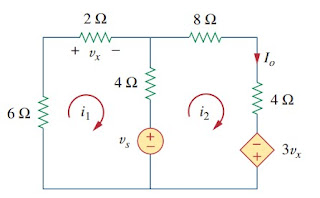Figure 2
Solution :
Applying KVL to the two loops, we get(1.1)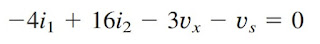(1.2)
But vx = 2i1. Equation (1.2) becomes(1.3)
Adding (1.1) and (1.3) we obtain
Substituting this with (1.1), we get
When vs = 12 V,
When vs = 24 V,
Showing that when the source value is doubled, Io doubles.

2. Assume Io = 1 A and use linearity to find the actual value of Io in the circuit of Figure.(3).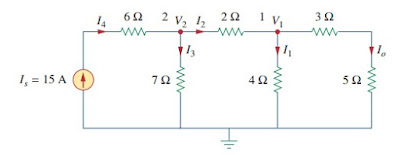Figure 3
Solution :
If Io = 1 A, then V1 = (3 + 5)Io = 8 V and I1 = V1/4 = 2 A. Applying KCL at node 1 gives
Applying KCL at node 2 gives
Therefore, Is = 5 A. This shows that assuming Io = 1 gives Is = 5 A, the actual source current of 15 A will give Io = 3 A as the actual value.

Untuk Bahasa Indonesia baca Teorema Rangkaian Listrik dan Rangkaian Linear.
Related Posts
SHARE